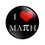# Help me out!

Here is a tremendously huge time consuming and awkward looking question: $(x+y+z)^3=(y+z-x)^3+(z+x-y)^3+(x+y-z)^3+kxyz$ $\text{Find}\space k.$

I have tried to take $(y+z-x)^3$ to the Left hand side and tried to use $a^3-b^3=(a-b)(a^2+b^2+ab)$, but I just kept on revolving and revolving and ended up being more confused than before.

Can anybody help me out by showing me how to factorize the expression or show me a simpler way?

$\huge\color{#D61F06}\text{Just a 12 year old math loving kid.}$ $\mathbb{\huge{\color{#456461}{\text{\#ASIMPLERWAY}}}}$

I am stuck in an another question, that is:

If $x,y$ and $z$ are real and unequal numbers, prove that: $2015x^2+2015y^2+6z^2>2(2012xy+3yz+3xz)$Note by Aaryan Maheshwari
3 years, 10 months ago

This discussion board is a place to discuss our Daily Challenges and the math and science related to those challenges. Explanations are more than just a solution — they should explain the steps and thinking strategies that you used to obtain the solution. Comments should further the discussion of math and science.

When posting on Brilliant:

• Use the emojis to react to an explanation, whether you're congratulating a job well done , or just really confused .
• Ask specific questions about the challenge or the steps in somebody's explanation. Well-posed questions can add a lot to the discussion, but posting "I don't understand!" doesn't help anyone.
• Try to contribute something new to the discussion, whether it is an extension, generalization or other idea related to the challenge.

MarkdownAppears as
*italics* or _italics_ italics
**bold** or __bold__ bold
- bulleted- list
• bulleted
• list
1. numbered2. list
1. numbered
2. list
Note: you must add a full line of space before and after lists for them to show up correctly
paragraph 1paragraph 2

paragraph 1

paragraph 2

[example link](https://brilliant.org)example link
> This is a quote
This is a quote
    # I indented these lines
# 4 spaces, and now they show
# up as a code block.

print "hello world"
# I indented these lines
# 4 spaces, and now they show
# up as a code block.

print "hello world"
MathAppears as
Remember to wrap math in $$ ... $$ or $ ... $ to ensure proper formatting.
2 \times 3 $2 \times 3$
2^{34} $2^{34}$
a_{i-1} $a_{i-1}$
\frac{2}{3} $\frac{2}{3}$
\sqrt{2} $\sqrt{2}$
\sum_{i=1}^3 $\sum_{i=1}^3$
\sin \theta $\sin \theta$
\boxed{123} $\boxed{123}$

Sort by:

Substitute $x=y=z=1$ and you will get $k=24$.

To prove that it's an algebraic identity for $k=24$, let $X = y+z-x, Y = z+x-y, Z = x+y-z$, and use the algebraic identity $X^3 + Y^3 + Z^3 = 3XYZ + (X+Y+Z)(X^2+Y^2+Z^2-XY-YZ-XZ)$.

- 3 years, 10 months ago

- 3 years, 10 months ago

Find the coefficient of each term

$x^3: 1 = -1 + 1 + 1$
$x^2 y: 3 = 3 -3 +3$
$xyz: 6 = -6 - 6 - 6 + k$

Hence, $k = 24$ (and we can also conclude that we have an identity).

Staff - 3 years, 10 months ago

How can we find the coefficient?

- 3 years, 10 months ago

A bonus question:

$\text{If}\space a=2015, b=2014\space \text{and}\space c=\frac{1}{2014}, \text{prove that}$ $(a+b+c)^3-(a+b-c)^3-(b+c-a)^3-(c+a-b)^3-23abc=2015$

- 3 years, 10 months ago